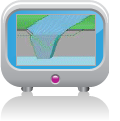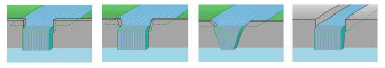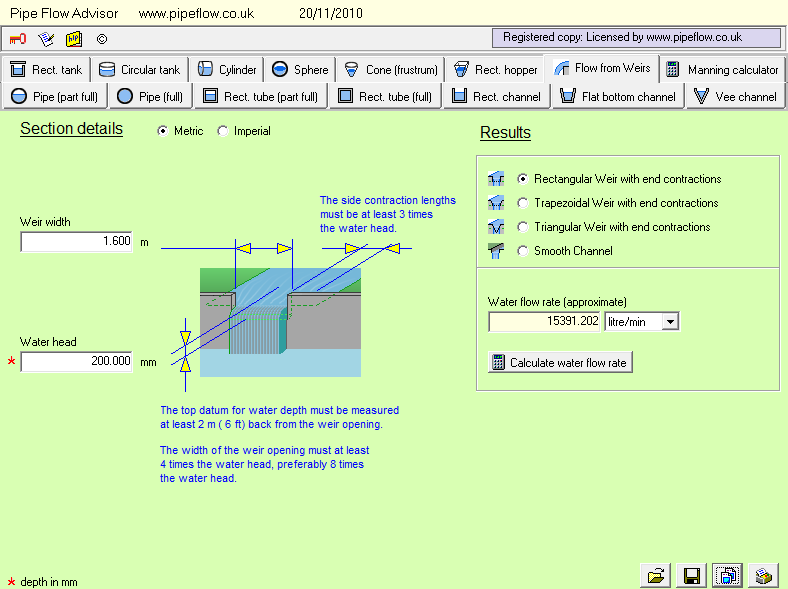# Weir Flow CalculatorThe flow rate of water over a weir can be estimated based on data related to the charactersitic of the weir and certain properties of the flow. Different shaped weirs require different flow calculations to estimate the weir's volumetric flow rate. Pipe Flow Advisor can calculate the estimated flow rate for these types of weir:• Rectangular weir with end contractions.
• Trapezoidal weir with sloping end contactions.
• Triangular weir with end contractions.
• Smooth channel (horizontal) without contactions.

## Weir Flow Calculation Screen

Pipe Flow Advisor allows you to select the type of weir design for which you need to calculate the flow rate. Different shapes of weir require their own specific calculation method to estimate the flow rate that will occur.

Pipe Flow Advisor can perform four different calculations, using the dimensions of the weir, the weir's water head (raised to a specific power, dependent on the design of weir), and a constant factor (also related to the specific design of weir under consideration).There is a different calculation screen for each type of Weir:

Select a type of weir design (Rectangular Weir, Trapezoidal Weir, Triangular Weir, Smooth Channel Weir without contractions).
Enter the weir width and weir water head data.

Click to calculate the estimated flow rate over the weir.

Next: Types of Tank

Secure Online PaymentsPipe Flow Software: Piping design, Pressure drop calculator, Flow rate calculator, Pump head calculations, Pump selection software.  Copyright © Pipe Flow Software 1997-2023